Double-sweep method

A method for transferring a one-point boundary condition by means of a differential or difference equation corresponding to the given equation. It is used for solving boundary value problems when the shooting method is ineffective.

Suppose one is given, on an interval, the linear ordinary differential equation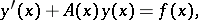(1)

where the square matrixhas order,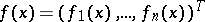is a vector of known continuous functions, and where the differentiable vector functionhas to be determined. Boundary conditions of the form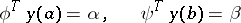(2)

are added to (1). Here,andare known matrices of dimension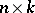and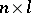and rankand, respectively,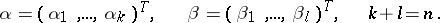By using the differential equations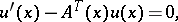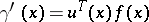with initial conditions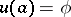,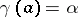, where the unknown differentiable matrix function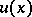has dimensionand, one can determineand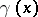on the whole interval(direct double-sweep). Using the equation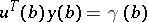and the second equation of (2) one can determine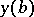, if the square matrix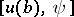has rank. The unknown solution of the boundary value problem (1)–(2) can now be computed as the solution of the Cauchy problem for (1) in the direction from the point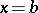to the point(inverse double-sweep). The method indicated is also applicable to multi-point problems, when constraints of the form (2) are given not only at the end points, but also at some interior points of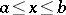. Versions of the double-sweep method for transferring linear boundary conditions different from (2) have been developed (cf. ).

The merit of the double-sweep method is obvious in the following boundary value problem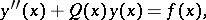(3)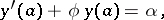(4)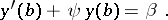(5)

Here,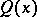is a square matrix of order,is a vector of dimensionof known continuous functions, the twice-differentiable vector function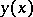is to be determined,andare known square matrices of order,, and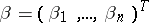. By using the differential equations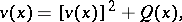with initial conditions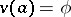,, one can determine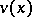andon the whole interval(direct double-sweep). Here,is a differentiable square matrix of orderand.

Using the equations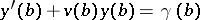and (5) one can determine(6)

if the matrix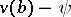has rank. The required solution of the boundary value problem (3)–(5) is the solution of the Cauchy problem for the equation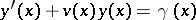with initial condition (6) (inverse double-sweep). Thus, the double-sweep method for (3)–(5) is a method lowering the order of the differential equation (3).

In the case of a finite sequence of linear algebraic equations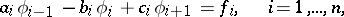(7)

where the coefficients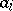,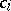andare known square matrices of orderand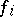,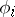are the known and required column-vectors of dimension,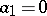,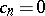, the double-sweep algorithm can be defined as follows: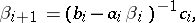(8)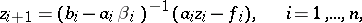(9)

under the conditions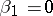,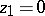(direct) and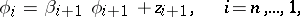(10)

under the condition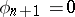(inverse). Here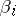is a square matrix of orderand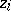,are column-vectors of dimension. The method indicated is called right double-sweep. Analogous to (8)–(10) one obtains the formulas for left double-sweep. By combining left and right double-sweeps, one obtains the method of meeting double-sweep. In solving (7) with variable coefficients one applies the preparatory double-sweep method. In order to find periodic solutions of an infinite sequence of equations of the form (7) with periodic coefficients one uses cyclic double-sweep (cf. ).